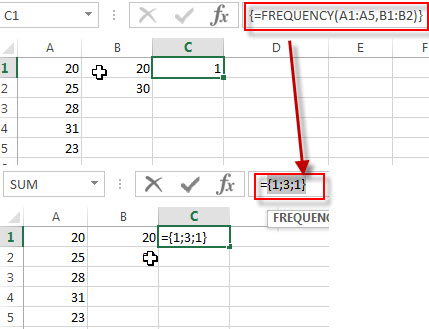# Excel FREQUENCY Function

This post will guide you how to use the FREQUENCY function with syntax and examples in Microsoft excel.

### Description

The Excel FREQUENCY function calculates how often values occur within a range of values. And it will return a vertical array of numbers. For example, you can use the FREQUENCY function to get the number of scores that fall within ranges of scores.

The FREQUENCY function is a build-in function in Microsoft Excel and it is categorized as a Statistical Function.

The FREQUENCY function is available in Excel 2016, Excel 2013, Excel 2010, Excel 2007, Excel 2003, Excel XP, Excel 2000, Excel 2011 for Mac.

### Syntax

The syntax of the FREQUENCY function is as below:

`= FREQUENCY (data_array, bins_array)`

Where the FREQUENCY function arguments are:

• Data_array -This is a required argument.  An array or reference or range of values for which you want to count the frequencies. And if the data_array do not contain any values, then the FREQUENCY function will return an array of zeros.
• bins_array – This is a required argument.  An array or reference or range of values of intervals that you want to group the values in data_array. And if bins_array do not contain any values, the FREQUENCY function will return the number of elements in data_array.

Note:

• If you want to return array result, then you need to the FREQUENCY formula as Array formula. When you create array formula, you need to press Ctrl + Shift + Enter on the formula box. And you will see that it will create brackets {} around your formula. You should know that if there is no brackets around your formula, it will not be treated as an array formula.
• After you select a range of adjacent cells into which you want to return distribution, and the FREQUENCY need to be entered as an array formula.
• The FREQUENCY function will ignore blank cells and contain text.

### Excel FREQUENCY Function Examples

The below examples will show you how to use Excel FREQUENCY Function to get the frequency of values in a data set.

1# to count the frequency of values in a data set, with data_array A1:A5, and bins_array B1:B2 , using the following array formula (you must be entered as an array formula via pressing Ctrl + Shift + Enter shortcuts):

`{=FREQUENCY(A1:A5,B1:B2)}`